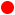MODEL: RCV-3000 Infrared USB ASCII Output Table The RCV-3000 Infrared Receiver standard version is programmed to recognize six of the most popular infrared code protocols used in infrared remote controls: NEC, RC5, Sony, Mitsubishi. Toshiba and Matsushita. The RCV-3000 will transmit ASCII strings based on the infrared code protocol. The following table describes the ASCII output format. The RCV-3000 can be customized to receive other infrared protocols and transmit custom ASCII or HEX data. Infrared Protocol ASCII Sample Description and notesNEC NXXYYZZ "N" identifies the string as NEC protocol "XXYY" represents the System Code "ZZ" represents the Command Code Example: N6E9100, where the System Code is "6E91" and the Command Code is "00". The NEC protocol may also use a "simple repeat" code for a key that is held down (indicates the same infrared code is being sent repeatedly). The simple repeat format is: "N!", where "N" is the NEC identifier and "!" is the simple repeat command. Example "simple repeat" for key being pressed and held: N6E9100 N! N! N! etc.RC5 RTXYY "R" identifies the string as RC5 protocol "TX" represents the System Code, with "T" being the RC5 toggle bit "YY" represents the Command Code Example: R0A00, where 0A is the System Code (and the toggle will be 2A) and "00" is the Command Code. The first digit of the System Code is also used as the toggle bit. The RC5 Protocol uses the toggle bit to distinguish a single key press from a key that is held down (and continues to issue infrared codes). With each single key press and release of the same key: R0A00 R2A00 R0A00 R2A00 (the RC5 toggle bit in this example switches between 0 and 2) If the same key is pressed and held: R0A00 R0A00 R0A00 etc. (note the toggle bit does not change).Sony SXXYY "S" identifies the string as Sony protocol "XX" represents the System Code "YY" represents the Command Code Example: S3A00, where "3A" is the System Code and "00" is the Command Code.Mitsubishi BXXYY "B" identifies the string as Mitsubishi protocol "XX" represents the System Code "YY" represents the Command Code Example: B3A00, where "3A" is the System Code and "00" is the Command Code.Toshiba TXXYYZZ "T" identifies the string as Toshiba protocol "XXYY" represents the System Code "ZZ" represents the Command Code Example: T6E9100, where the System Code is "6E91" and the Command Code is "00".Matsushita MXXYY "M" identifies the string as Matsushita protocol "XX" represents the System Code "YY" represents the Command Code Example: M3A00, where "3A" is the System Code and "00" is the Command Code.